# MCAT Physical : Distillation

## Example Questions

### Example Question #1 : Purification Techniques

Consider the following solutions.

Solution A: 1M sodium chloride solution

Solution B: 1M calcium nitrate solution

Solution C: 1M sucrose solution

Equal volumes of the solutions are combined and the mixture is added to a distillation column. Which of the following solutions will separate first?

Solution A

These solutions cannot be separated via distillation

Solution C

Solution B

Solution C

Explanation:

Distillation is a process of separating a liquid from solutes or other liquids. It utilizes the boiling point differences to separate substances. A substance with a low boiling point will evaporate first in a distillation column and will be isolated first. The question is asking which solution will be isolated first; therefore, we need to figure out which solution has the lowest boiling point. Recall that the boiling point of a solution is elevated when there are more solutes present in the solution. Sodium chloride (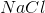) contributes two solutes (sodium ions and chloride ions). Calcium nitrate (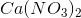) contributes three solutes (one calcium ion and two nitrate ions). Sucrose does not dissociate into ions in solution; therefore, it only contributes one solute. This means that the sucrose solution will have the lowest amount of molecules in solution, the lowest boiling point, and will be separated first.

### Example Question #2 : Purification Techniques

Small differences in boiling point require the use of __________ distillation and large differences in boiling point require the use of __________ distillation.

fractional . . . simple

simple . . . fractional

simple . . . simple

fractional . . . fractional

fractional . . . simple

Explanation:

There are two types of distillation. Simple distillation is used to separate molecules that have very different boiling points. Fractional distillation is used to separate molecules with small differences in boiling points. Fractional distillation is often used if the difference between boiling points is less than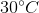. In simple distillation, the vapor is immediately collected in a condenser. On the other hand, fractional distillation allows vapor to condense and revaporize several times. These repeated cycles allow fractional distillation to purify the vapor better than simple distillation.

### Example Question #3 : Purification Techniques

Which of the following mixtures can be separated using fractional distillation (boiling points of each substance given in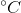)?

I. Chloroform (62.2) and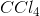(76.7)

II. Iodine (184.3) and mercury (356.9)

III. Nitric acid (120) and sulfuric acid (310)

II and III

II only

I, II, and III

I only

I, II, and III

Explanation:

Distillation is used to separate molecules with different boiling points. Simple distillation is used to separate molecules with vastly different boiling points. Fractional distillation, on the other hand, is a refined form of simple distillation that can be used to separate molecules with similar boiling points. Note that fractional distillation can separate molecules with either different or similar boiling points; therefore, fractional distillation can be used to separate any of the given mixtures.

### Example Question #4 : Purification Techniques

Which of the following conditions will result in the greatest increase in the rate of distillation of a substance?

Decreasing the vapor pressure

Decreasing the mole fraction of the substance

Decreasing the atmospheric pressure

Decreasing the temperature